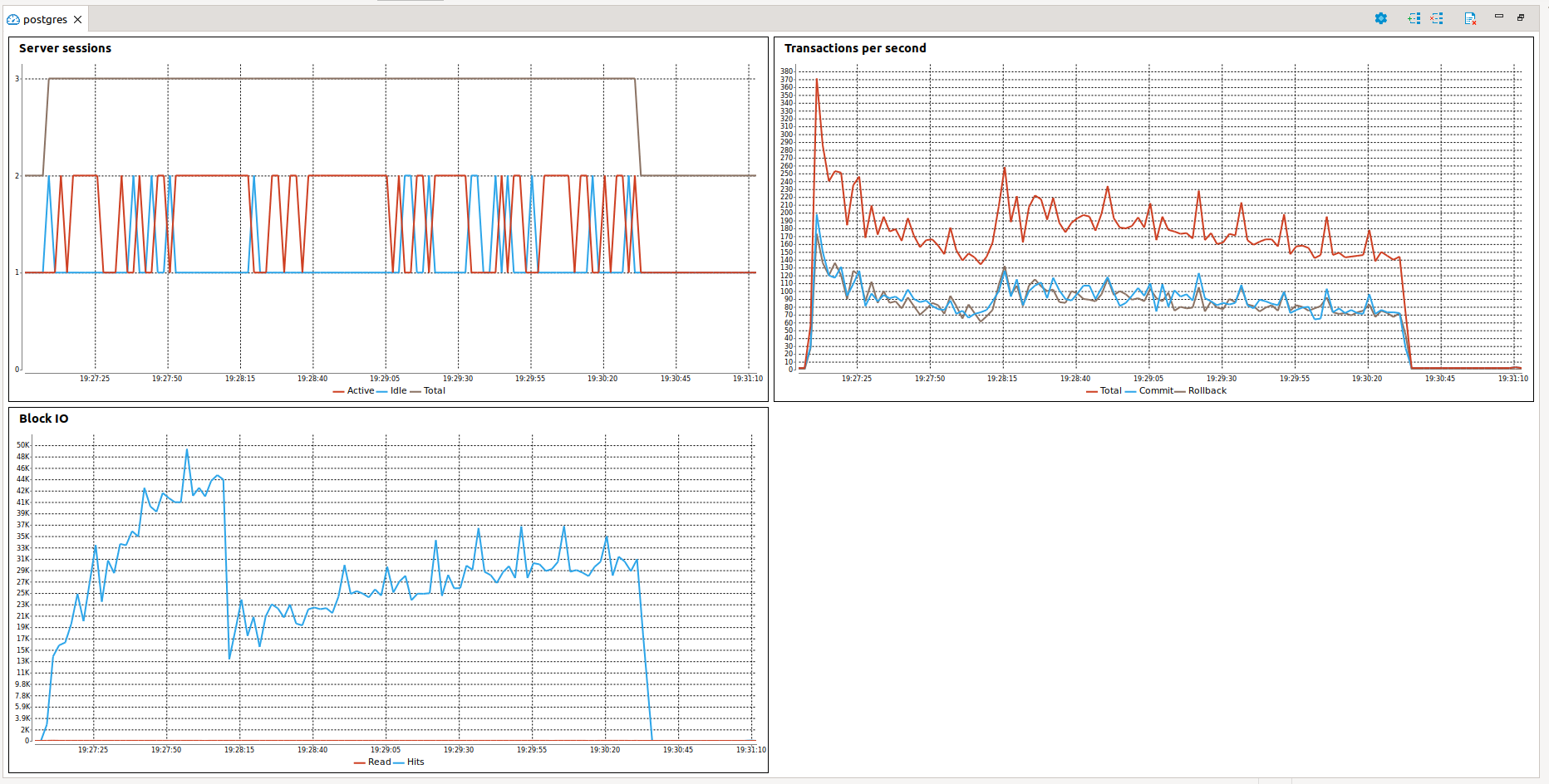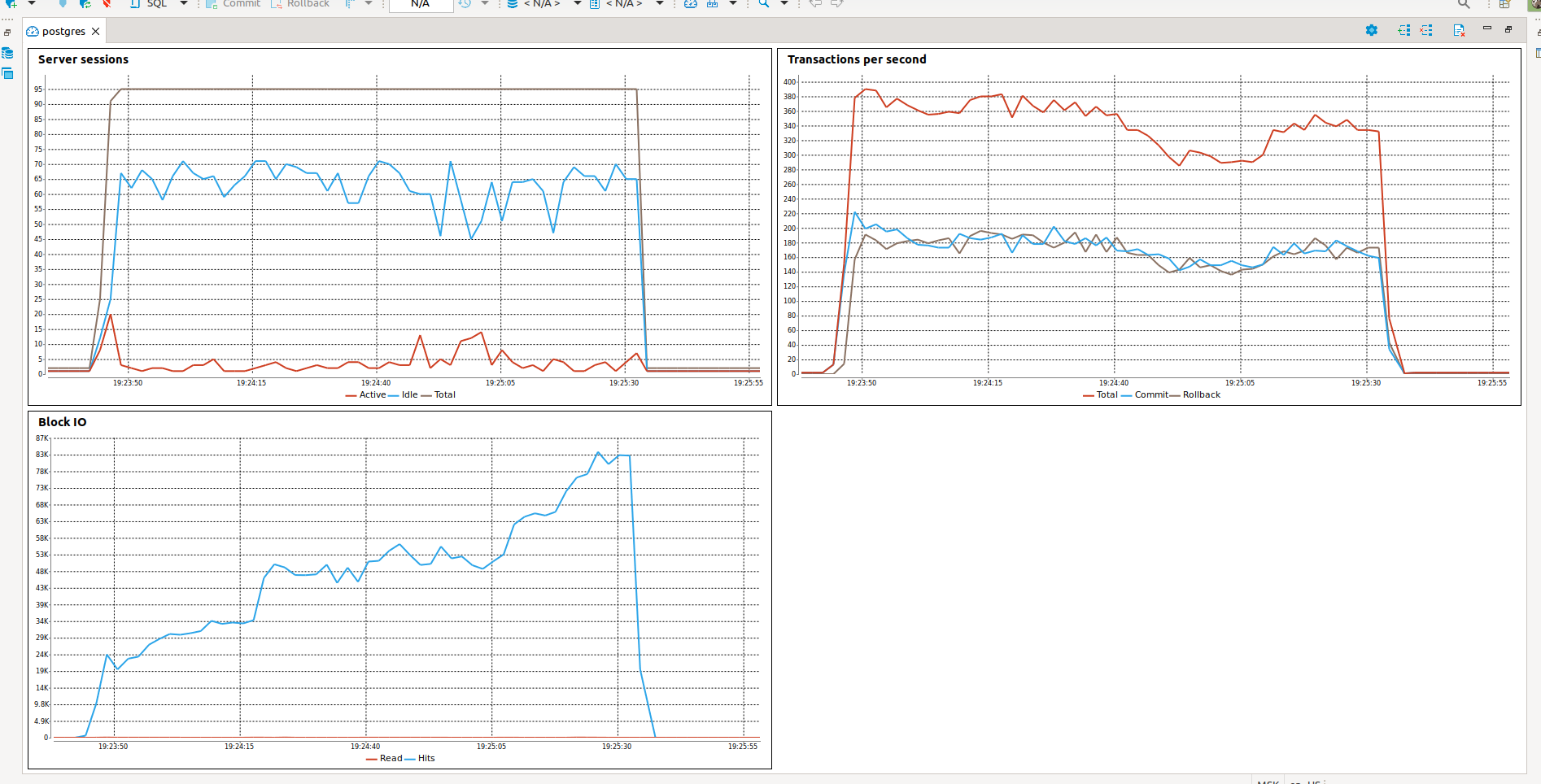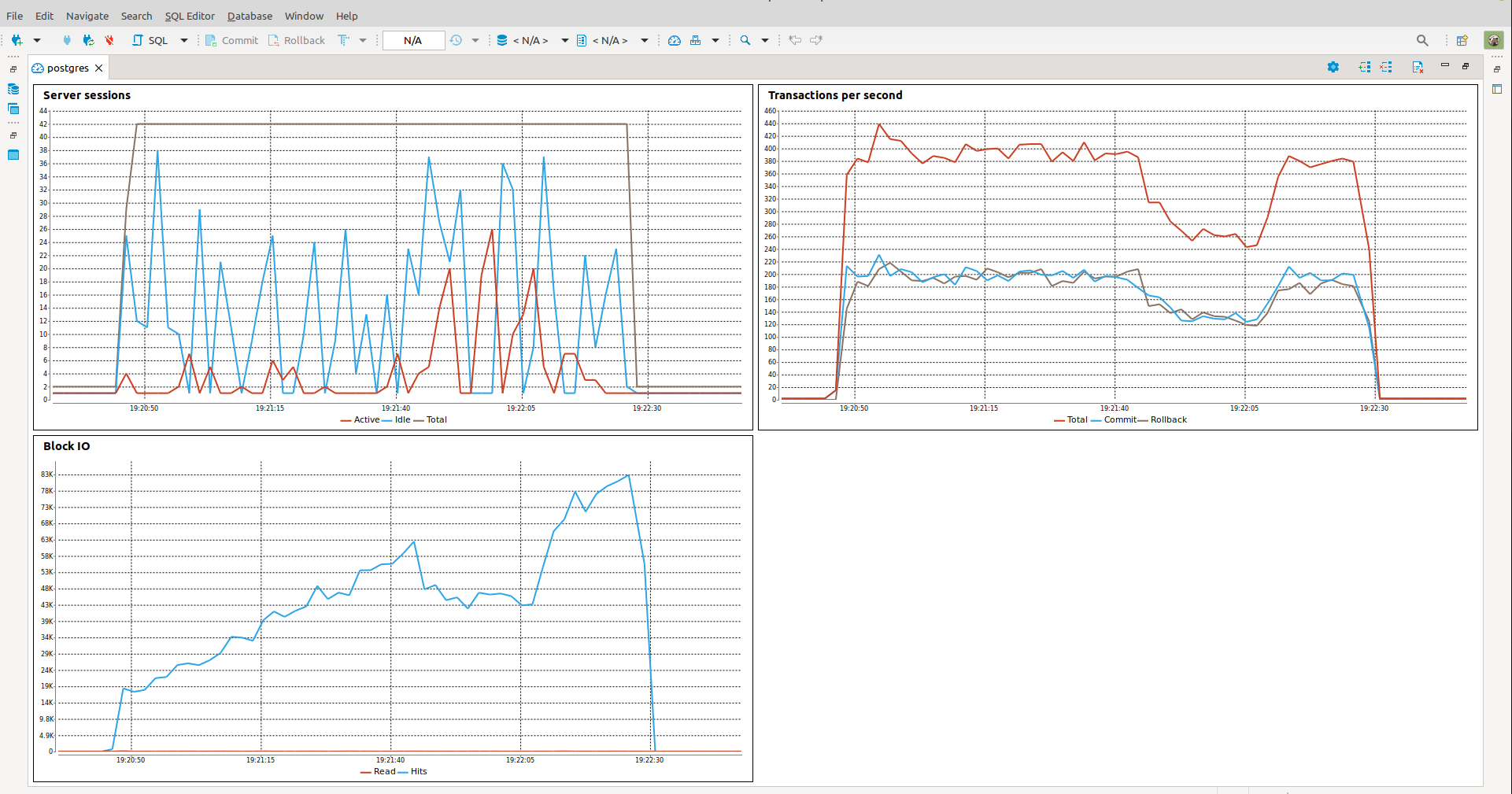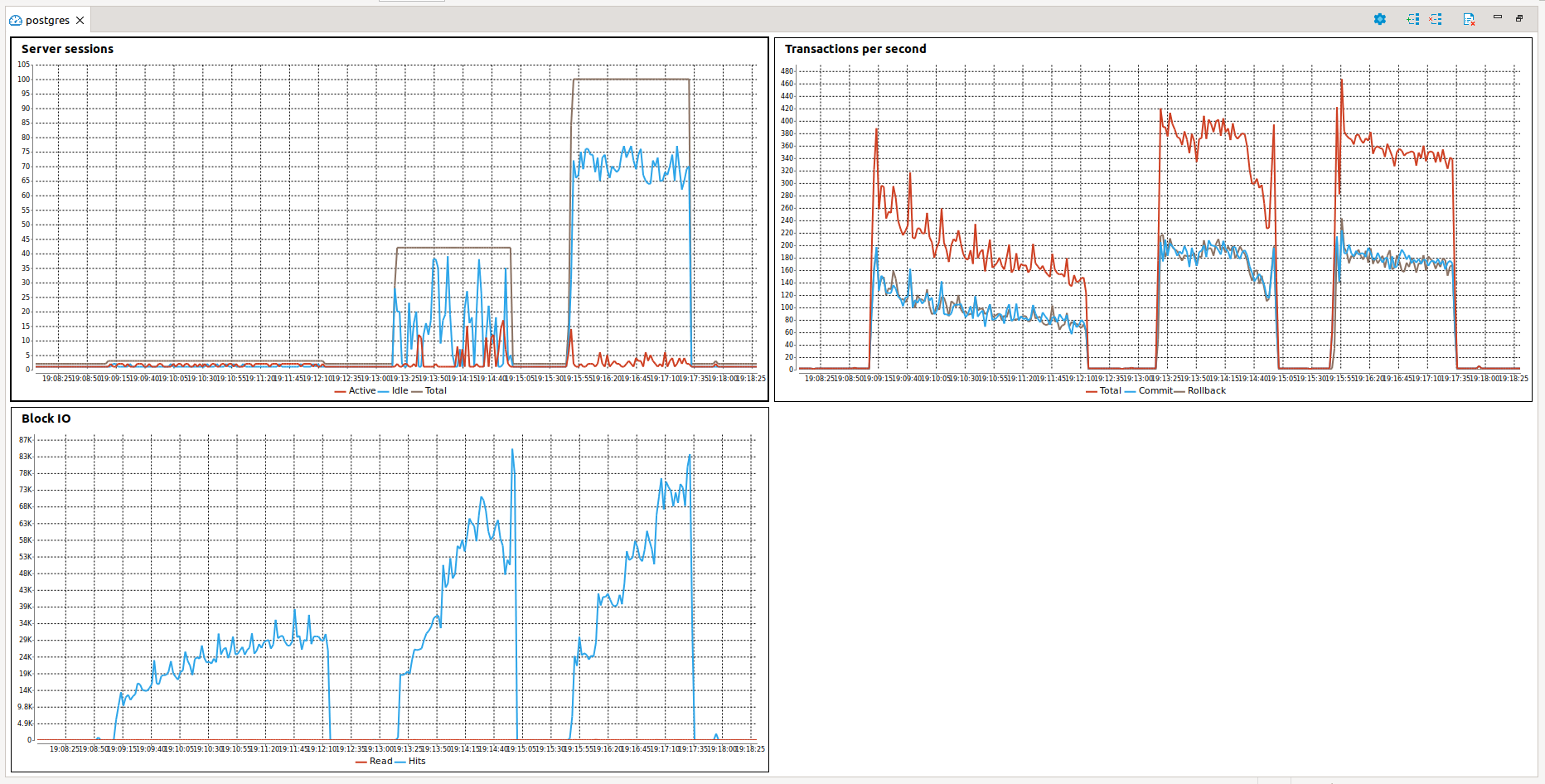From SQLAlchemy documentation since SQLAlchemy release 1.4 there present some support of async I/O: SQLAlchemy docs

The new asyncio feature should be considered alpha level for the initial releases of SQLAlchemy 1.4. This is super new stuff that uses some previously unfamiliar programming techniques. The initial database API supported is the asyncpg asyncio driver for PostgreSQL.

By the latest docs I can see now Asynchronous I/O (asyncio)

The asyncio extension as of SQLAlchemy 1.4.3 can now be considered to be beta level software. API details are subject to change however at this point it is unlikely for there to be significant backwards-incompatible changes.

For today the current release is 1.4.31 and I have an idea to run some tests to look closer on it.

So first install virtual environment, install there SQLAlchemy, asyncpg driver and psycopg2-binary driver, I have an idea to do some tests with usual sync flow:

``````\$ python3.10 -m venv .venv
\$ source .venv/bin/activate

\$ (.venv) pip install sqlalchemy asyncpg psycopg2-binary

\$ pip freeze
asyncpg==0.25.0
greenlet==1.1.2
psycopg2-binary==2.9.3
SQLAlchemy==1.4.31
``````

Now we need postgresql database, run it with docker Docker, and set 2000 max_connections:

``````docker run --rm \
--name postgres \
-p 5432:5432 \
-e POSTGRES_USER=postgres \
-e POSTGRES_DB=postgres \
postgres -N 2000

...
2022-02-11 08:00:34.288 UTC  LOG:  starting PostgreSQL 14.1 (Debian 14.1-1.pgdg110+1) on x86_64-pc-linux-gnu, compiled by gcc (Debian 10.2.1-6) 10.2.1 20210110, 64-bit
``````

We can check if -N 2000 was applied:

``````docker run -it --rm --link postgres:postgres postgres psql -h postgres -U postgres;
psql (14.1 (Debian 14.1-1.pgdg110+1))
Type "help" for help.

postgres=# show max_connections ;
max_connections
-----------------
2000
(1 row)
``````

So all is fine, our patient is prepared and waits for our commands.

### The case

I think about to run ~35000 read/write operations against database, it should be ~ 50/50 %. ~50% read, 50% write operations. And its interesting to look at total time of execution and how many connections were utilized.

### Sync story

I prepared some code that in my opinion does what I want in style I used to do usually: file sync_main.py

``````import random
import time
from functools import partial

from sqlalchemy import Column
from sqlalchemy import DateTime
from sqlalchemy import ForeignKey
from sqlalchemy import func
from sqlalchemy import Integer
from sqlalchemy import String

from sqlalchemy import create_engine
from sqlalchemy.orm import Session

from sqlalchemy.future import select
from sqlalchemy.orm import declarative_base
from sqlalchemy.orm import relationship
from sqlalchemy.orm import sessionmaker

Base = declarative_base()

class A(Base):
__tablename__ = "a"

id = Column(Integer, primary_key=True)
data = Column(String)
create_date = Column(DateTime, server_default=func.now())
bs = relationship("B")

class B(Base):
__tablename__ = "b"
id = Column(Integer, primary_key=True)
a_id = Column(ForeignKey("a.id"))
data = Column(String)

def init_session():
engine = create_engine(
"postgresql+psycopg2://postgres:postgres@localhost/postgres",
echo=False, pool_size=2000, max_overflow=0, pool_timeout=60
)
session = sessionmaker(engine, future=True)
return session

def create_entities(session, data):
with session() as session:
with session.begin():
[
A(bs=[B(), B()], data=str(data + 1)),
A(bs=[B()], data=str(data + 2)),
A(bs=[B(), B()], data=str(data + 3)),
]
)

with session() as session:
result = session.execute(stmt)
for a1 in result.scalars():
for b1 in a1.bs:
print(b1)

def sync_main():
"""Main program function."""
session = init_session()
create_entities_list = list()
for i in range(35000):
if not random.choice([True, False]):
else:
create_entities_list.append(partial(create_entities, session, random.randint(1, 100_000)))
t()
print('created: ', len(create_entities_list))

if __name__ == '__main__':
start = time.time()
sync_main()
print('Total time: ', time.time()-start)
``````

Here we have 2 models with relationship, I prepared two lists of operations, then concat them in one big list and shuffle tasks, to have random order. Result:

`````` created:  17565
Total time:  203.5927267074585
``````

in that case total time is ~203 seconds and look at screenshot of DBeaver database dashboard:The DBeaver itself uses database connections, so in total we can see 3 connections on graph, I suppose two of them its DBeaver’s connections. So I may be wrong, but here we utilize single connection from database for execute our flow syncronously.

### Async story one

Check docs about questions how to use in whole SQLAlchemy asyncio extension, in addition there many articles were written about it, so its boring to repeat docs.

``````import asyncio
import itertools
import random
import time

from sqlalchemy import Column
from sqlalchemy import DateTime
from sqlalchemy import ForeignKey
from sqlalchemy import func
from sqlalchemy import Integer
from sqlalchemy import String

from sqlalchemy.ext.asyncio import AsyncSession
from sqlalchemy.ext.asyncio import create_async_engine
from sqlalchemy.future import select
from sqlalchemy.orm import declarative_base
from sqlalchemy.orm import relationship
from sqlalchemy.orm import sessionmaker

Base = declarative_base()

class A(Base):
__tablename__ = "a"

id = Column(Integer, primary_key=True)
data = Column(String)
create_date = Column(DateTime, server_default=func.now())
bs = relationship("B")

# required in order to access columns with server defaults
# or SQL expression defaults, subsequent to a flush, without
__mapper_args__ = {"eager_defaults": True}

class B(Base):
__tablename__ = "b"
id = Column(Integer, primary_key=True)
a_id = Column(ForeignKey("a.id"))
data = Column(String)

async def init_session():
engine = create_async_engine(
"postgresql+asyncpg://postgres:postgres@localhost/postgres",
future=True, echo=False, pool_size=3000, max_overflow=20, pool_timeout=60
)

async with engine.begin() as conn:
async with engine.begin() as conn:
# expire_on_commit=False will prevent attributes from being expired
# after commit.
async_session = sessionmaker(
engine, expire_on_commit=False, class_=AsyncSession
)
return async_session

async def coro_create_entities(async_session, data):
async with async_session() as session:
async with session.begin():
[
A(bs=[B(), B()], data=str(data + 1)),
A(bs=[B()], data=str(data + 2)),
A(bs=[B(), B()], data=str(data + 3)),
]
)

async with async_session() as session:
# for streaming ORM results, AsyncSession.stream() may be used.
result = await session.stream(stmt)
# result is a streaming AsyncResult object.
async for a1 in result.scalars():
for b1 in a1.bs:
print(b1)

async def async_main():
"""Main program function."""
async_session = await init_session()
create_entities = set()

for i in range(35000):
if not random.choice([True, False]):
else:
coro_create_entities(
async_session, data=random.randint(1, 100_000))))
await asyncio.sleep(0)  # trick to let switch context from creating tasks

done, pending = await asyncio.wait(

print("Done: ", len(done))
print("Pending: ", len(pending))

print('created: ', len(create_entities))

if __name__ == '__main__':
start = time.time()
asyncio.run(async_main())
print('Total time: ', time.time()-start)
``````

And result here is amazing !!! The total time is two times faster than in case with sync style:

``````Done:  35000
Pending:  0
created:  17663
Total time:  116.1717700958252
``````It utilizes ~100 database connections. The one thing you can see my trick that lets my script to switch attention to task execution from ‘for’ loop that pushes tasks to event loop queue. Otherwise, if I don’t do that, our 2000 pool will be exhausted by 2000 tasks in 3 seconds.

### Async story two (chunks)

Here I decided to push tasks by chunks, to control optimal numbers of concurrent requests per unit of time.

``````import asyncio
import itertools
import random
import time

from sqlalchemy import Column
from sqlalchemy import DateTime
from sqlalchemy import ForeignKey
from sqlalchemy import func
from sqlalchemy import Integer
from sqlalchemy import String

from sqlalchemy.ext.asyncio import AsyncSession
from sqlalchemy.ext.asyncio import create_async_engine
from sqlalchemy.future import select
from sqlalchemy.orm import declarative_base
from sqlalchemy.orm import relationship
from sqlalchemy.orm import sessionmaker

from itertools import zip_longest

def chunker(iterable, chunksize, filler):
return zip_longest(*[iter(iterable)]*chunksize, fillvalue=filler)

Base = declarative_base()

class A(Base):
__tablename__ = "a"

id = Column(Integer, primary_key=True)
data = Column(String)
create_date = Column(DateTime, server_default=func.now())
bs = relationship("B")

# required in order to access columns with server defaults
# or SQL expression defaults, subsequent to a flush, without
__mapper_args__ = {"eager_defaults": True}

class B(Base):
__tablename__ = "b"
id = Column(Integer, primary_key=True)
a_id = Column(ForeignKey("a.id"))
data = Column(String)

async def init_session():
engine = create_async_engine(
"postgresql+asyncpg://postgres:postgres@localhost/postgres",
future=True, echo=False, pool_size=3000, max_overflow=20, pool_timeout=60
)

async with engine.begin() as conn:
async with engine.begin() as conn:

# expire_on_commit=False will prevent attributes from being expired
# after commit.
async_session = sessionmaker(
engine, expire_on_commit=False, class_=AsyncSession
)
return async_session

async def coro_create_entities(async_session, data):
async with async_session() as session:
async with session.begin():
[
A(bs=[B(), B()], data=str(data + 1)),
A(bs=[B()], data=str(data + 2)),
A(bs=[B(), B()], data=str(data + 3)),
]
)

async with async_session() as session:
# AsyncSession.execute() is used for 2.0 style ORM execution
# (same as the synchronous API).
# for streaming ORM results, AsyncSession.stream() may be used.
result = await session.stream(stmt)
# result is a streaming AsyncResult object.
async for a1 in result.scalars():
for b1 in a1.bs:
print(b1)

async def async_main():
"""Main program function."""
async_session = await init_session()
create_entities_list = list()

for i in range(35000):
if not random.choice([True, False]):
else:
create_entities_list.append(coro_create_entities(
async_session, data=random.randint(1, 100_000)))

for i, chunk in enumerate(chunker(tasks, 40, None)):
await asyncio.gather(*(t for t in chunk if t))

print('created: ', len(create_entities_list))

if __name__ == '__main__':
start = time.time()
asyncio.run(async_main())
print('Total time: ', time.time()-start)
``````

So as you can see from code I push by forthy requests (Tasks) per one chunk and we can see results:

``````created:  17600
Total time:  102.18556618690491
``````

so here less total time of execution, I win 14 seconds !!! Its because I think its less load on database when I control chunks of tasks to run concurrently at one loop cycle. And of course DBeaver picture:### The end

So final words

scenario time, sec
sync 202.0
async 116.0
async chunked 102.2

And here you can look at all three runs on one graph.I hope it was interesting, feel free to comment below ;)